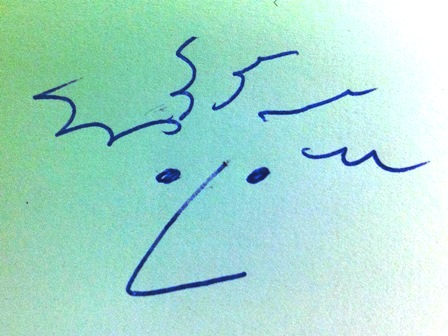## Paul Gröneveld @ Fab Academy 2016 >>> Embedded programming

<<<< go back

The lecture on this topic was interesting and difficult for me at the same time because it is very new to me. The goal of this week is reading the micro controller data sheet and programming the board that was made two weeks ago.

In the video below the production of a CPU is explained.

In this case we specifically look at the ATtiny24/44/84 CPU. The picture below shows the pins from the 84 smd version.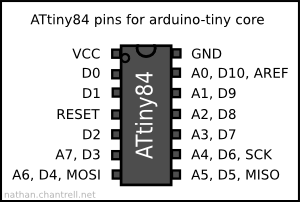The main architecture of the chip is explained in the block schematic below. On the boundery of the box all pins are coming out including VCC and GND.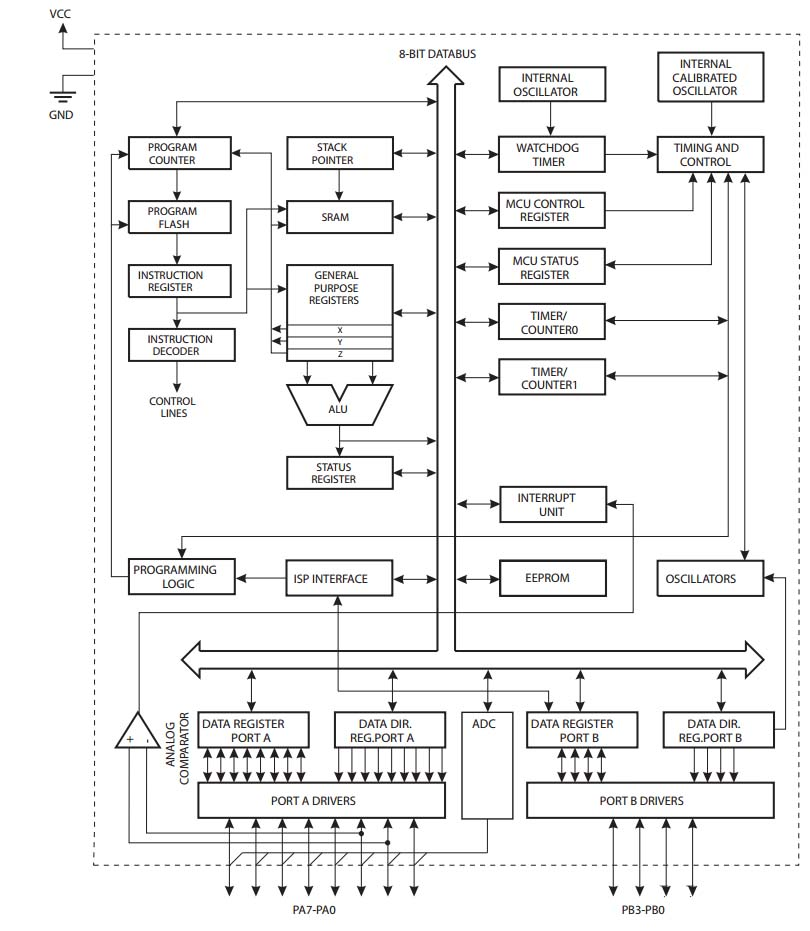Below the block diagram of the AVR-Architecture is displayed.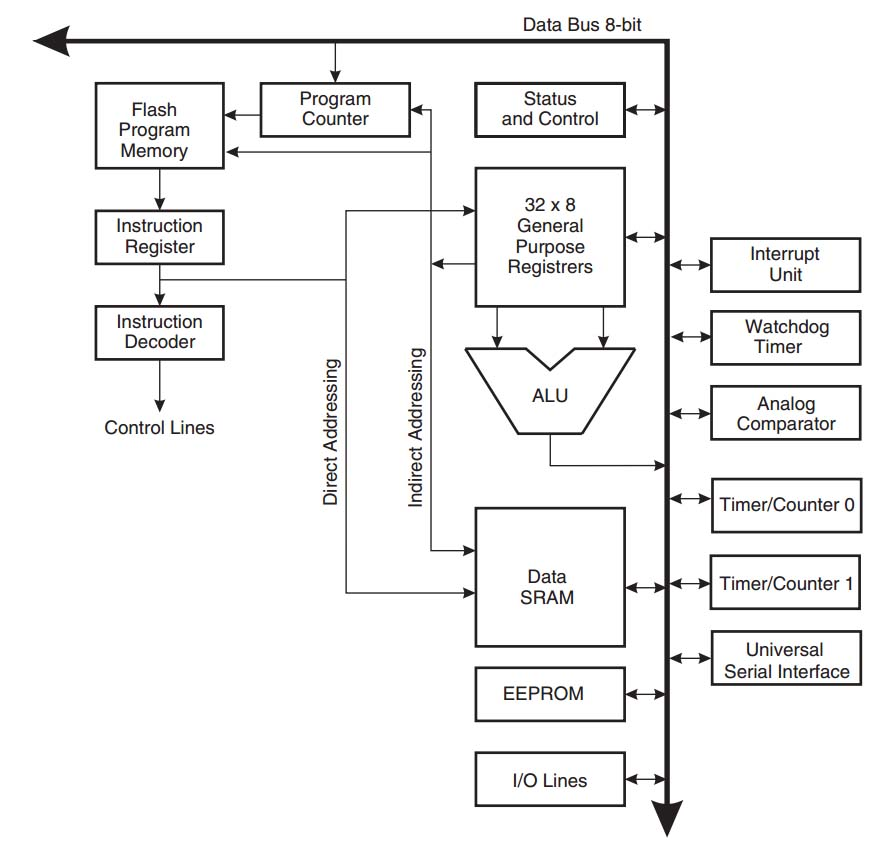Reading the datasheet gave an insight on many different subjects on square millimeters. Often many details that I could not really understand. .......

# Programming the board

When we started working with my first board it became clear that there was a problem with the IC. Replacing the IC was too complicated because there were too many problems in it already. According to Emma it was a big mess and I agree so I decided to make a new board. For details see the end of week 6.

## Hardware

We now have three devices, a computer in this case a laptop with Windows 7, an In Circuit Programmes FabTiny*ISP that was made in week 4 and the new board with 3 switches and an RGB-led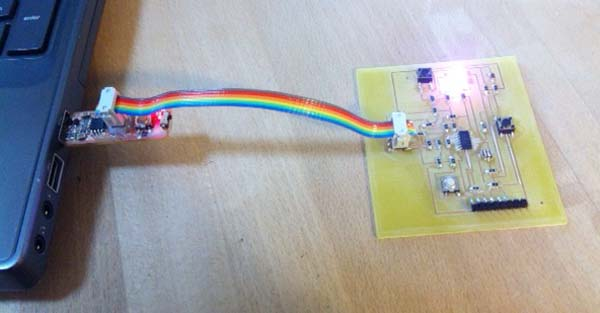Before we are able to work with the board different software and drivers had to be installed. Below the different steps are shown.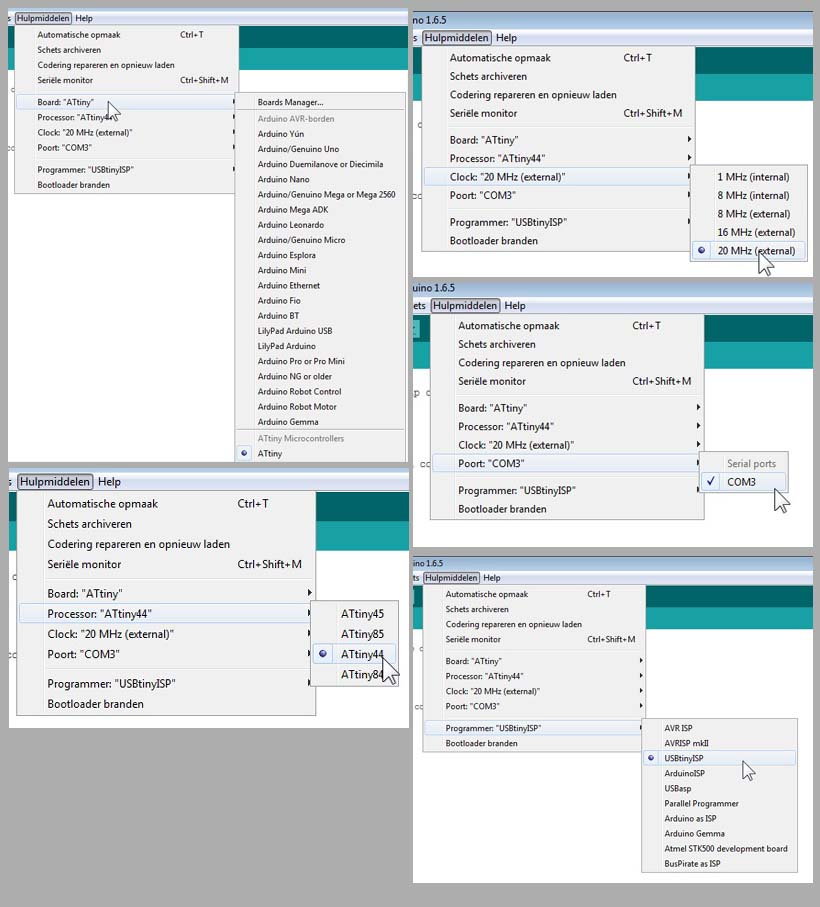Basically you are ready for uploading and testing the code on the board after all these steps but first it is wise to check if communication with the board through the ISP is possible. Therefore connect the devices. To do this, open the command prompt (cmd) and type the following command: avrdude -c usbtiny -p t44 (hit enter). The reaction from this should be a confirmation that the device is ready to accept instructions. A signature from the device is shown (8 digit code) and a safe mode that the fuses are ok. The picture below shows the result of the check.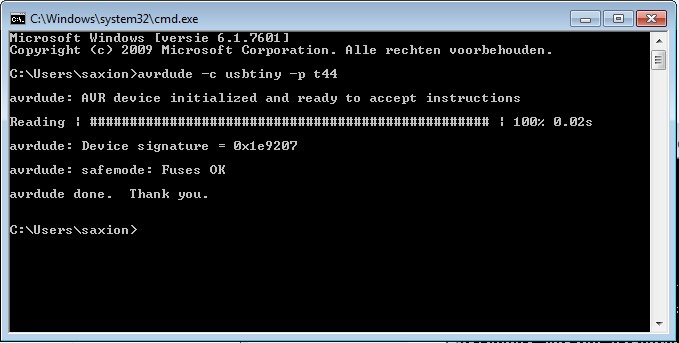The conclusion here is that communication with the board is possible.

# Pin connections on the board

To find out the connections the schematic of the board has to be compared with the datasheet from the IC and the configuration that the Arduino IDE uses. Below The schematic and the board schematic is presented.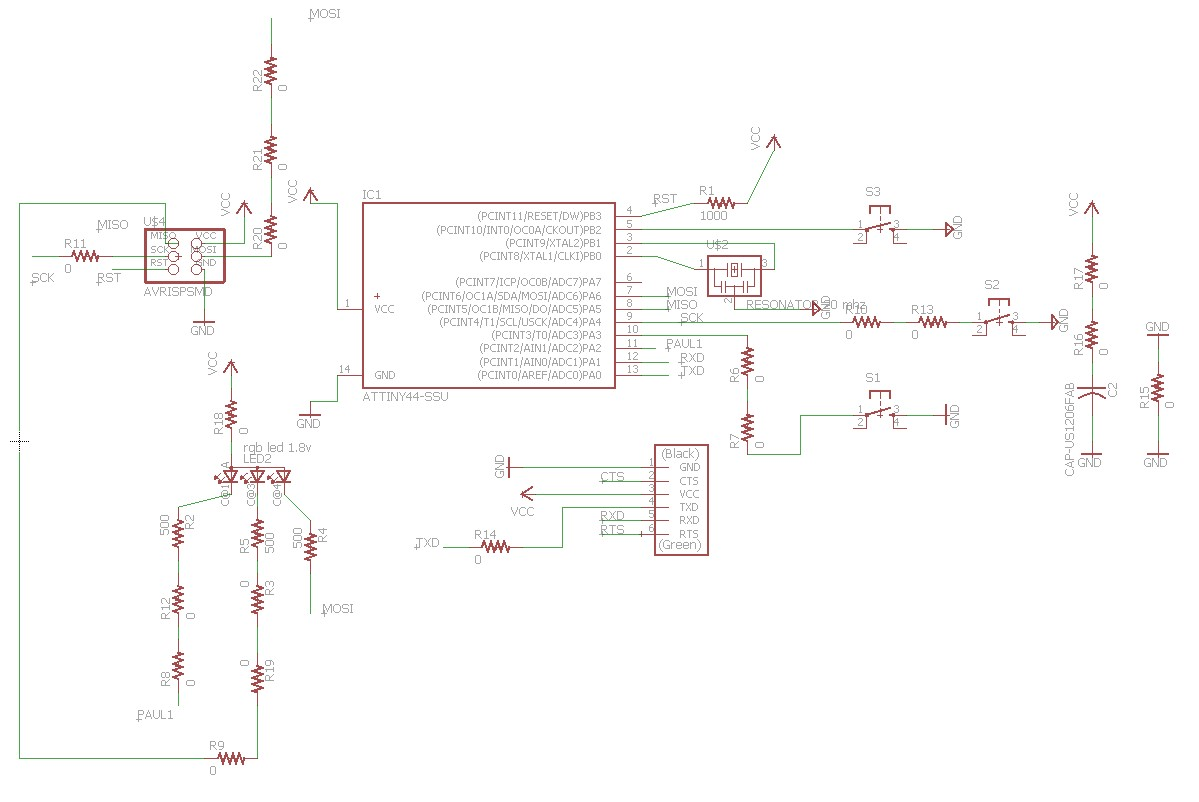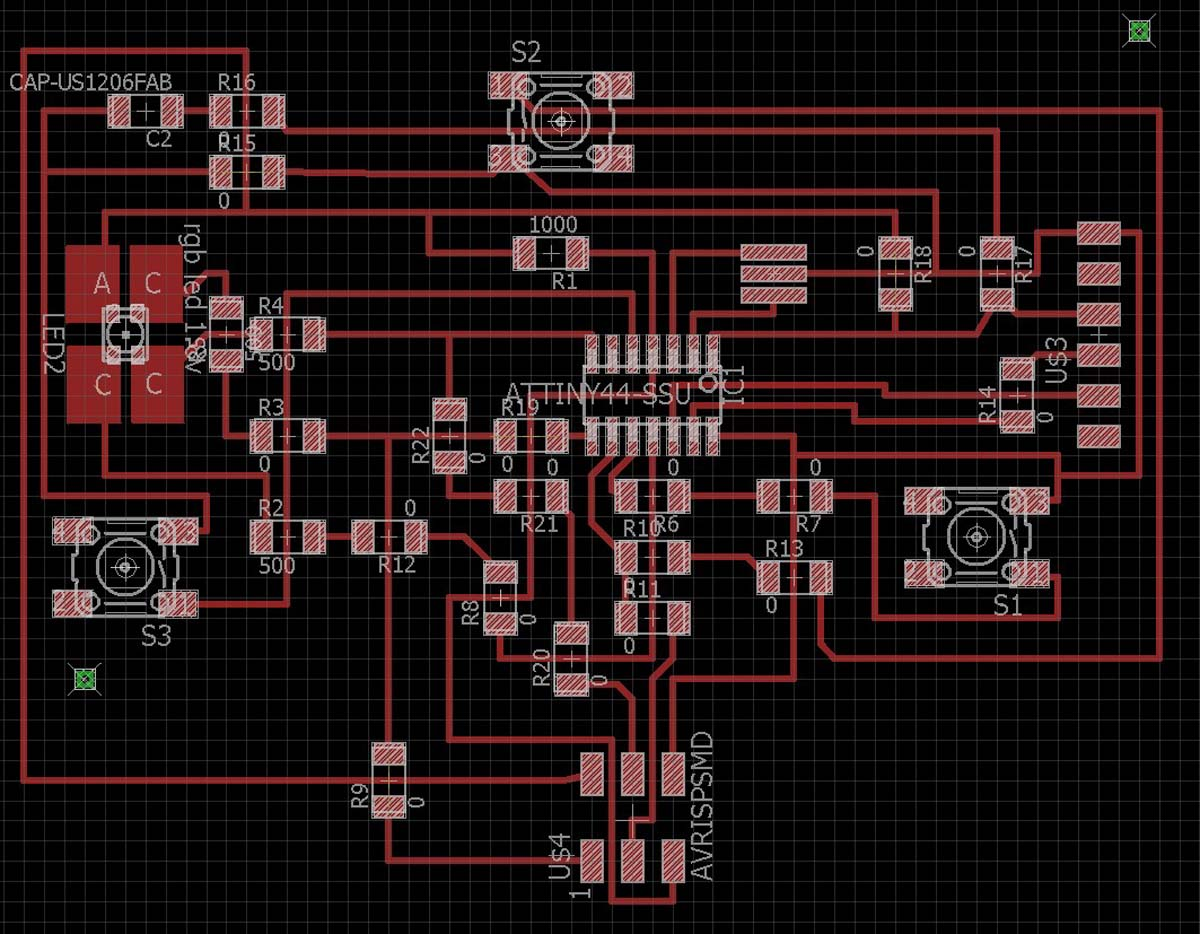With the help of the schematic, the board layout, the data sheet from the IC and the arduino data sheet the right pin names can be determined.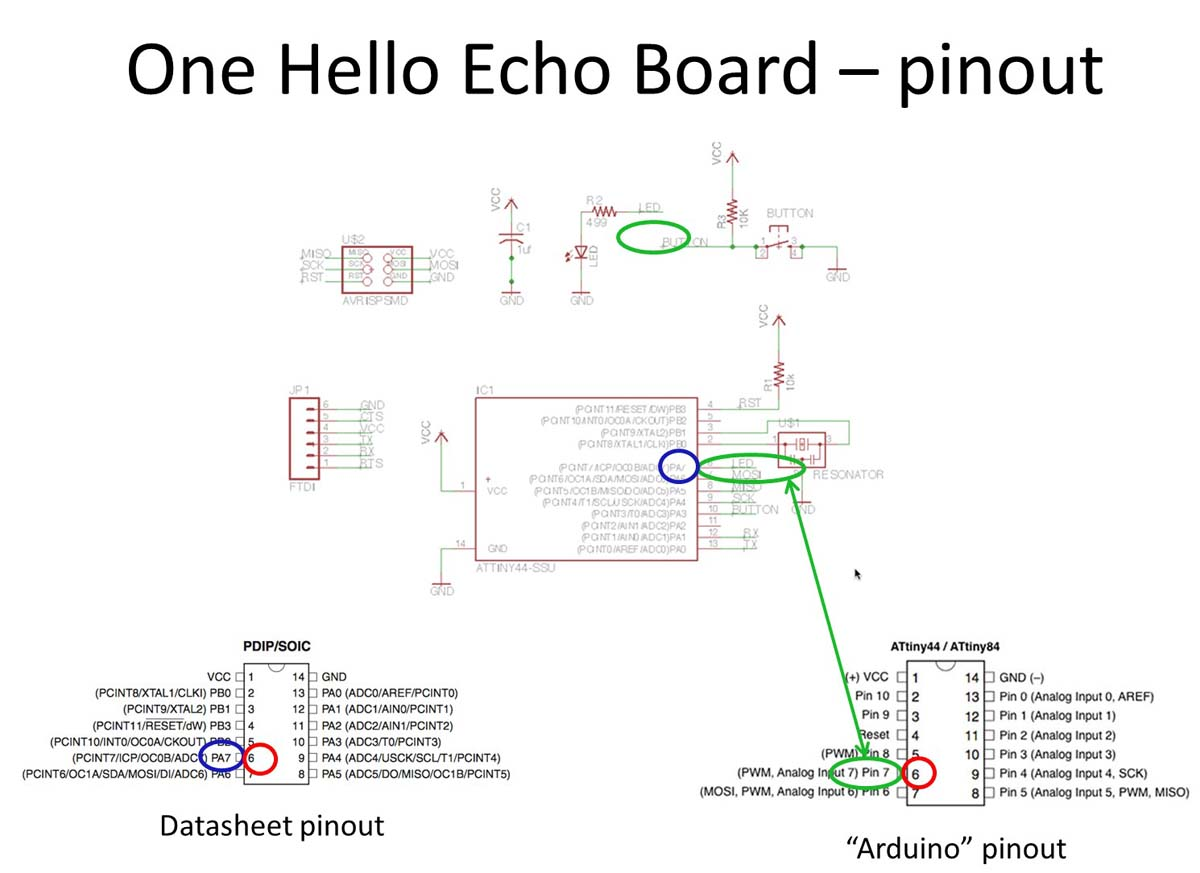## Pin connections.

How to find the right pin names to use in the adruino code? First check in the schedule how the Led is connected. Write down the name of the connection. In the IC other names are used. Transfer the line name to the PA or PB code. Use the PA or PB code to find the right number in the drawing from the chip in the data sheet. This last number should be transformed to the "Arduino" pin output for the same chip.

Led 1 > PAUL1 > 11 > PA2 > 11 > PIN2 > Blue

Led 2 > MISO > 8 > PA5 > 8 > PIN5 > Red

Led 3 > MOSI > 7 > PA6 > 7 > PIN6 > Green

Switch 1 > ??? > 10 > PA3 > 10 > PIN3

Switch 2 > PAUL1 > 9 > PA4 > 9 > PIN4

Switch 3 > ??? > 5 > PB2 > 5 > PIN8

# Programming the board

Before you start programming you should first "burn the boat loader". This is kind of a reset of the chip so it can be programmed. Also later this command can be used, if there are problems with uploading sketches (code).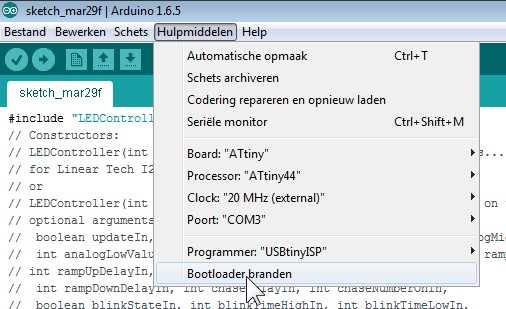This week I had many problems with uploading the program to the board. Many times I get an error which often results in a program that is not running well. The error is as follows: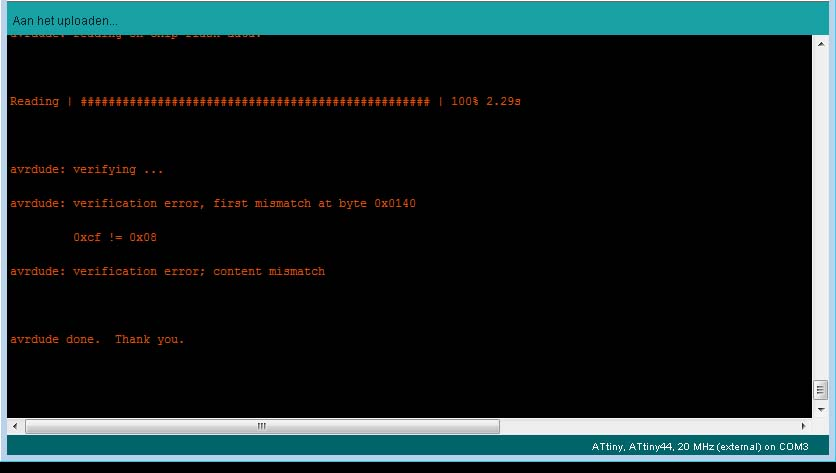On my own and together with people from the Fabacademy I tried many things to solve this, like: (1) Use another ISP, (2) Use an other board, (3) use another ISP and Board, (4) use another computer (Mac), (5) use a new desktop Windows 7 PC, (6) Reinstalling all software on my laptop. This all resulted in not solving the problem. It could not be reproduced stable so no conclusions could be taken.

By all this testing I discovered that eventually it will be possible to upload the program. This can be done by; closing Arduino IDE and restarting, and/or, disconnecting the ISP and reconnecting the ISP, and/or, try another program first. This all took a lot of time..... In the beginning I did not know how to check the errors......Now I would recommend to change the settings and make the black screen bigger.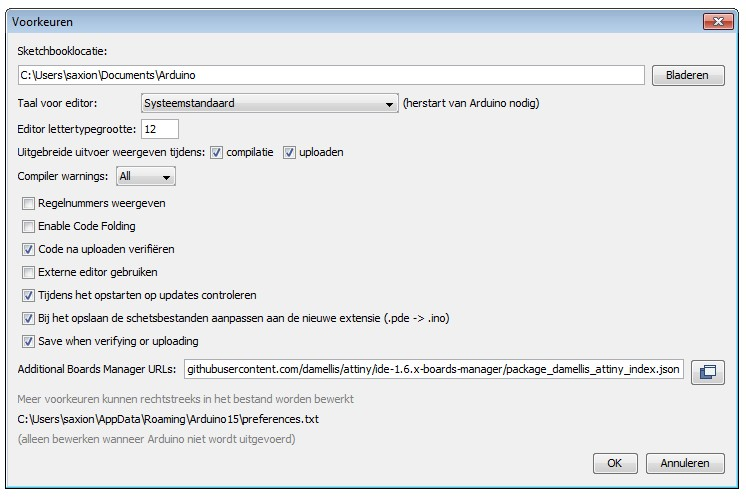# Testing different programs

Below different sketches (codes) for Arduino are tested. Each time the video shows the result. On the right the conclusion of the test is given. The orange conclusions still have problems.

# Testing different programs

Below different sketches (codes) for Arduino are tested. Each time the video shows the result. On the right the conclusion of the test is given. The orange conclusions still have problems.

 ```void setup() {  // initialize digital pin 13 as an output.  pinMode(2, OUTPUT); } // the loop function runs over and over again forever void loop() {  digitalWrite(2, HIGH);   // turn the LED on (HIGH is the voltage level)  delay(1000);              // wait for a second  digitalWrite(2, LOW);    // turn the LED off by making the voltage LOW  delay(1000);              // wait for a second } ``` Simple blinking bue led. Conclusion: blinking blue led works well.

 ```void setup() {  // initialize digital pin 13 as an output.  pinMode(5, OUTPUT); } // the loop function runs over and over again forever void loop() {  digitalWrite(5, HIGH);   // turn the LED on (HIGH is the voltage level)  delay(1000);              // wait for a second  digitalWrite(5, LOW);    // turn the LED off by making the voltage LOW  delay(1000);              // wait for a second } ``` Simple blinking red led. Conclusion: blinking red led works well.

 ```void setup() {  // initialize digital pin 13 as an output.  pinMode(6, OUTPUT); } // the loop function runs over and over again forever void loop() {  digitalWrite(6, HIGH);   // turn the LED on (HIGH is the voltage level)  delay(200);              // wait for a second  digitalWrite(6, LOW);    // turn the LED off by making the voltage LOW  delay(200);              // wait for a second ``` Simple blinking green led with shorter interval. Conclusion: blinking green led works well.

 ```// constants won't change. They're used here to // set pin numbers: const int buttonPin = 3;     // the number of the pushbutton pin const int ledPin =  2;      // the number of the LED pin // variables will change: int buttonState = 0;         // variable for reading the pushbutton status void setup() {  // initialize the LED pin as an output:  pinMode(ledPin, OUTPUT);  // initialize the pushbutton pin as an input:  pinMode(buttonPin, INPUT); } void loop() {  // read the state of the pushbutton value:  buttonState = digitalRead(buttonPin);  // check if the pushbutton is pressed.  // if it is, the buttonState is HIGH:  if (buttonState == HIGH) {    // turn LED on:    digitalWrite(ledPin, HIGH);  }  else {    // turn LED off:    digitalWrite(ledPin, LOW);  } } ``` Standaard button program from Arduino IDE. Conclusion: Not good. Light is already on and not reaction well enough to button.

 ```const int buttonPin1 = 3;     // the number of the pushbutton pin const int ledPin1 =  2;      // the number of the LED pin // variables will change: int buttonState1 = 0;         // variable for reading the pushbutton status void setup() {  // initialize the LED pin as an output:  pinMode(ledPin1, OUTPUT);  // initialize the pushbutton pin as an input:  pinMode(buttonPin1, INPUT_PULLUP); } void loop() {  // read the state of the pushbutton value:  buttonState1 = digitalRead(buttonPin1);  // check if the pushbutton is pressed.  // if it is, the buttonState is HIGH:  if (buttonState1 == HIGH) {    // turn LED on:    digitalWrite(ledPin1, HIGH);  }  else {    // turn LED off:    digitalWrite(ledPin1, LOW); ``` Modified button program. Conclusion: With : "INPUT_PULLUP" on the buttonpin the switch works well. The voltage on this pin was not stable

 ```// constants won't change. They're used here to // set pin numbers: const int buttonPin1 = 4;     // the number of the pushbutton pin const int ledPin1 =  5;      // the number of the LED pin // variables will change: int buttonState1 = 0;         // variable for reading the pushbutton status void setup() {  // initialize the LED pin as an output:  pinMode(ledPin1, OUTPUT);  // initialize the pushbutton pin as an input:  pinMode(buttonPin1, INPUT_PULLUP); } void loop() {  // read the state of the pushbutton value:  buttonState1 = digitalRead(buttonPin1);  // check if the pushbutton is pressed.  // if it is, the buttonState is HIGH:  if (buttonState1 == HIGH) {    // turn LED on:    digitalWrite(ledPin1, HIGH);  }  else {    // turn LED off:    digitalWrite(ledPin1, LOW); } } ``` Buttonswitch 2, connected to red led. Conclusion: Red led is constant on, this is not good. Led does not react to buttoinswitch. Reason unknown.

 ```// constants won't change. They're used here to // set pin numbers: const int buttonPin1 = 8;     // the number of the pushbutton pin const int ledPin1 =  6;      // the number of the LED pin // variables will change: int buttonState1 = 0;         // variable for reading the pushbutton status void setup() {  // initialize the LED pin as an output:  pinMode(ledPin1, OUTPUT);  // initialize the pushbutton pin as an input:  pinMode(buttonPin1, INPUT_PULLUP); } void loop() {  // read the state of the pushbutton value:  buttonState1 = digitalRead(buttonPin1);  // check if the pushbutton is pressed.  // if it is, the buttonState is HIGH:  if (buttonState1 == HIGH) {    // turn LED on:    digitalWrite(ledPin1, HIGH);  }  else {    // turn LED off:    digitalWrite(ledPin1, LOW); } } ``` Buttonswitch 3, connected to green led. Conclusion: Switch 3 works well on green led.

 Tutorial RGB led. ```int r_pin = 2; int g_pin = 5; int b_pin = 6; void setup() {  // put your setup code here, to run once: pinMode(r_pin,OUTPUT); pinMode(g_pin,OUTPUT); pinMode(b_pin,OUTPUT); } void loop() {  // put your main code here, to run repeatedly: digitalWrite(r_pin,LOW); digitalWrite(g_pin,HIGH); digitalWrite(b_pin,HIGH); delay(1000); digitalWrite(g_pin,LOW); digitalWrite(b_pin,HIGH); digitalWrite(r_pin,HIGH); delay(1000); digitalWrite(b_pin,LOW); digitalWrite(g_pin,HIGH); digitalWrite(r_pin,HIGH); delay(1000); } ``` RGB blinking after eachother. Conclusion: RBG blinks very nice after eachother

 Tutorial RGB fading led.------------------------------------------------```int r_pin = 2; int g_pin = 5; int b_pin = 6; int r_val = 0; int g_val = 255; int b_val = 255; void setup() { pinMode(r_pin,OUTPUT); pinMode(g_pin,OUTPUT); pinMode(b_pin,OUTPUT); } void loop() { while (r_val != 255) {  analogWrite(r_pin,r_val);  analogWrite(g_pin,g_val);  r_val++;  g_val--;  delay(20); } while (g_val != 255) {  analogWrite(g_pin,g_val);  analogWrite(b_pin,b_val);  g_val++;  b_val--;  delay(20); } while (b_val != 255) {  analogWrite(b_pin,b_val);  analogWrite(r_pin,r_val);  b_val++;  r_val--;  delay(20); } } ``` RGB-fluent fading into eachother Conclusion: One pint is not suitable for PWM, this result in not fluently fading of the led.

 ```int r_pin = 2; int g_pin = 5; int r_val = 0; int g_val = 255; void setup() { pinMode(r_pin,OUTPUT); pinMode(g_pin,OUTPUT); } void loop() { while (r_val != 255) {  analogWrite(r_pin,r_val);  analogWrite(g_pin,g_val);  r_val++;  g_val--;  delay(20); } while (g_val != 255) {  analogWrite(g_pin,g_val);  analogWrite(r_pin,r_val);  g_val++;  r_val--;  delay(20); } } ``` Fading 2 colours. Conclusion: Pin 5 and 6 have PWM and fade nice together.

 ```int r_pin = 2; int g_pin = 5; int r_val = 0; int g_val = 255; void setup() { pinMode(r_pin,OUTPUT); pinMode(g_pin,OUTPUT); } void loop() { while (r_val != 255) {  analogWrite(r_pin,r_val);  analogWrite(g_pin,g_val);  r_val++;  g_val--;  delay(20); } while (g_val != 255) {  analogWrite(g_pin,g_val);  analogWrite(r_pin,r_val);  g_val++;  r_val--;  delay(20); } } ``` Leds op pin 2 en 5 faden. Conclusion: Pin 2 is not a PWM pin, therefore it is not fading fluently

 ```int r_pin = 2; int g_pin = 6; int r_val = 0; int g_val = 255; void setup() { pinMode(r_pin,OUTPUT); pinMode(g_pin,OUTPUT); } void loop() { while (r_val != 255) {  analogWrite(r_pin,r_val);  analogWrite(g_pin,g_val);  r_val++;  g_val--;  delay(20); } while (g_val != 255) {  analogWrite(g_pin,g_val);  analogWrite(r_pin,r_val);  g_val++;  r_val--;  delay(20); } } ``` Leds op pin 2 en 5 faden. Conclusion: Pin 2 is not a PWM pin, therefore it is not fading fluently

 ```} // the loop function runs over and over again forever void loop() {  digitalWrite(2, HIGH);   // turn the LED on (HIGH is the voltage level)  delay(0);              // wait for a second  digitalWrite(2, LOW);    // turn the LED off by making the voltage LOW  delay(0);              // wait for a second  digitalWrite(5, HIGH);   // turn the LED on (HIGH is the voltage level)  delay(0);              // wait for a second  digitalWrite(5, LOW);    // turn the LED off by making the voltage LOW  delay(0);              // wait for a second digitalWrite(6, HIGH);   // turn the LED on (HIGH is the voltage level)  delay(100);              // wait for a second  digitalWrite(6, LOW);    // turn the LED off by making the voltage LOW  delay(100);              // wait for a second ``` White light flashing with colour. Conclusion: RBG leds together generate white light. Blinking one colour creates a combined colour.

 ```//#include #include #define rxPin 0 #define txPin 1 SoftwareSerial serial(rxPin, txPin); void setup() {  pinMode(rxPin, INPUT);  pinMode(txPin, OUTPUT);  serial.begin(9600); } void loop() {  serial.println("Hallo Paul, this is your board!");  delay(1000); } ``` Reading what is happening with the FTDI serial cable. Conclusion: Reading what is happening works fine at a delay of 1 sec. It also works for 0.1 and 0.02 sec.

 ```int r_pin = 8; int g_pin = 5; int b_pin = 6; int r_val = 0; int g_val = 255; int b_val = 255; void setup() { pinMode(r_pin,OUTPUT); pinMode(g_pin,OUTPUT); pinMode(b_pin,OUTPUT); } void loop() { while (r_val != 255) {  analogWrite(r_pin,r_val);  analogWrite(g_pin,g_val);  r_val++;  g_val--;  delay(20); } while (g_val != 255) {  analogWrite(g_pin,g_val);  analogWrite(b_pin,b_val);  g_val++;  b_val--;  delay(20); } while (b_val != 255) {  analogWrite(b_pin,b_val);  analogWrite(r_pin,r_val);  b_val++;  r_val--;  delay(20); } } ``` !!!!RGB FADING Conclusion: I connected the problem LED to pin 8, and disconnected it from pin 2 by using a jump wire on the board.

# Reflection

Understanding what is going on inside a small programmable Chip is not easy. Many settings can be changed. Output pins and input pins have different properties. Soma are only digital and some are only analogue. Digital pins can be used as analogue pins by using WPN. For programming I only used Arduino, because that was already quite difficult to understand. Small programs are easy to understand. When it becomes more complex more knowledge about programming is needed. I assume programming in C for instance is basically the same, but probably more compact. Also here more knowledge about programming is needed. By using the serial FTDI-cable the results from the processor can be read and logged. I was looking for more advanced reading programs and found some, but was not able to adapt it to my board